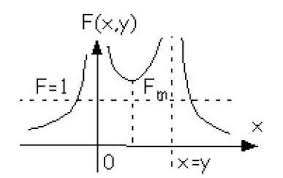## Monday, November 28, 2016

### A Closer Analytical Look At Two Stream InstabilityProfile for two-stream instability.Recall that in posts earlier this year we examined the two stream instability of plasma physics, which also has significance for solar and space physics.

We saw it can be induced by an energetic particle stream injected in a plasma, or setting a current along the plasma so different species (ions and electrons) can have different drift velocities. The energy from the particles can lead to plasma wave excitation.

The two stream instability can be thought of as the inverse of Landau damping, where a greater number of particles that move slower than the wave phase velocity vph (as compared with those that move faster), leads to an energy transfer from the wave to the particles.  In the case of the two stream instability, when an electron stream is injected to the plasma, the particle's velocity distribution function has a "bump" on its tail as shown in Fig. 1.

Development:

The electron plasma frequency can be written:

w e     =  [ne e2/ me  εo] ½

Where ne is the electron number density, e  is the electron charge,  me  is the electron mass and εo  is the permittivity of free space.

For our purposes in looking at the 2-stream instability we will change the above form to read:

w e2     =   4n o e2/ me

Similarly, we can write:

w i2     =   4n o e2m i

For the electron ion frequency.

For our purposes also, the Poisson equation, for charges in a vacuum:

Ñ ·E  =  4p r

Can be rewritten:

ik E =  4p r

Now, write   in terms of E such that:

ik (E -  4p r / ik ) = ikε E = 0

For cold plasma waves (T e =Ti  = 0)  we can write:

ik (1 -     w e 2 / w 2  ) E =  0

So that: ε    = (1 -     w e 2 / w 2  )

The dispersion relation here is equivalent to equating ε ( w)  to 0.

N.B.   A dispersion relation implies that a relationship exists between the plasma frequency w and the wave number k.

Now,  and this is critical, because we have ε ( w)  = 0 this implies:

1 =      w e 2 / w 2

Or:   w  =   w e

Now,  assembling all the preceding results allows us to write:

ik = 4p e [ (ik n o e)/ m iw 2    -   ik n o e/me (w  - k Vo ) 2]

Using the earlier equation for the electron and ion plasma frequencies and basic algebra, the energetic reader can satisfy himself that:

ε ( w)  =  1 -  w i  2 / w 2   -  w e 2 /(w  - k vo )=  0

Note that in the limit, m i    -> oo  and  w i -> 0  we have: w  =   k Vo  +  w e

We can then look at wave numbers k such that  k Vo  =   w e

and acknowledge that that 2nd term above is much less than unity (to cancel the 1st term the 3rd must be close to unity) whence:

0 =  1 -  w i  2 / w 2     -    w e 2 /(w  -   w e ) 2

0 =  1 -  w i  2 / w 2    -    1/(1  -   w  /  w e ) 2

0   »   1 -  w i  2 / w 2    -  (1 +     2w / w e )

And:  0 = -  w i  2 / w 2   -   2w / w e

w 3      =  - ½ ( w i ) 2  (w e)

Finally, we can write:

w /w e  =   ( -1/2) 1/3   (m e / m i) 1/3

which represents instability since one of the three values of  (-1) 1/3   is (1/2) + iÖ 3/ 2.

Thus, in the frame moving with the electrons, the Doppler shifted frequency (since   k Vo   w e) is

w’ =   w  - k Vo

Factor into the mix that |  w  |  < <  w e    and we conclude this is roughly w’ =   w e  so the electrons are nearly at their natural frequency of oscillation. But there is another way to determine that the dielectric function, i.e.

ε ( w)  =  1 -  w i  2 / w 2   -  w e 2 /(w  - k vo )=  0

yields instability. This can be done via a simple algebraic manipulation, so we transpose the negative terms to the right hand side and let:

F(k,  w ) =  ε ( w)  -  1  =  w i  2 / w 2   +   w e 2 /(w  - k vo )

We can then plot the resulting function vs. the real frequency (at fixed wave number, k)  as depicted in my 2nd graph above.

Note where the line at unity intersects the graph of  F(k,  w)  at four different points. In other words, there are four real roots and no instability for the selected value of k. But...what happens when the central minimum of  F(k,  w)  occurs at a value greater than unity? Then, there are only two real roots .

To determine when this happens we determine when:   F(k,  w ) >  1, or where the function minimum is determined by F/  w  =    0.  We then will find:

w  (min)  = (m e /m i) 1/2  k Vo

Yielding an equation which predicts instability whenever:

| k Vo  |    <   w e

I leave it to energetic readers to work out what that equation predicting instability will be.  (A  little bit of basic algebra will have you home free!)